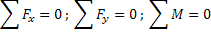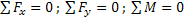# Assumptions and Analysis of Plain Truss

## Truss

A framework composed of straight members joined at their ends to form a structure to bear loads is called a truss. It is one of the major structures commonly used in engineering applications. It provides practical and economical solution to many engineering situations.

Trusses may be seen in steel bridges, supporting structures of roofs, electrical transmission towers, roller coasters and giant wheels of amusement parks and in innumerable other constructions.

Actually, most structures are made up of several trusses joined together to form a space framework. Each truss is designed to carry loads which act in its plane and thus may be treated as a two dimensional structure and can be considered as a plane truss for purpose of analysis.

The figures above are the various trusses in used to support various structures.

The loading of truss is designed in such a way that all external forces are applied at the joints. If loads are applied elsewhere, it will introduce bending loads and a truss cannot resist bending loads.

The analysis of truss is the process of finding the forces in the members of a truss.

The truss is one of the major types of engineering structures.

It is a structure made up of straight members joined together at their ends by pin-joints, where it is loaded.

Each member of the truss is normally uniform in cross section along its length.

The truss provides great strength over large spans in the airy structures than more solid type of structures.

It also provides practical and economical solution.

A truss consisting of coplanar members (such as a truss on both sides of a bridge) is called plane truss.

If a truss is made up of non-coplanar members, it is known as space truss.

Structural model:

An idealized truss is termed as just-rigid, if on the removal of any of its members destroys its rigidity.

An elementary stable (just-rigid) structure with three members form a triangle.

A simple relation between number of joints (j) and number of members (m) would be

m = 2j – 3 (Perfect Truss)

m > 2j – 3 (Redundant Truss)

m < 2j – 3 (Deficient Truss)

Assumptions:

The following assumptions are made in the analysis of truss

• · Truss members are connected only at their ends
• · Truss members are connected by frictionless pins
• · Trusses are loaded only at the joints
• · The weight of the members may be neglected

The ends of trusses are usually bolted, riveted or welded to a gusset plate.

Gusset plate is an intermediate structural element where the analysis of forces and moments are complicated in nature.

The members of a truss are usually long and slender, and can withstand large axial loads but cannot resist moments or large lateral loads.

Free body diagram of Joints and Members

Each member of a truss is subjected to the force only at the ends.

Hence members of trusses are two force members.

In a two member force, the forces act along the straight line joining the points where the forces are applied as shown in figure.

The force which pulls the members or tends to increase the length (or elongate) is known as tensile force. The member experiencing tensile force is said to be in ‘Tension’.

Force which pushes the member of tends to decrease the length (or compress) is known as compressive force. The member withstanding the compressive force is known to be in ‘Compression’.

The nomenclature is to name the force from A to B. For example, the force at point A due to B can be named as FAB.

## Method of Joints:

Truss may be considered as a group of two force members connected at their ends by friction-less pins.

Since the entire truss as a rigid body is in equilibrium, each part is also considered to be in equilibrium i.e. each member of the truss must be in equilibrium and each joint of the truss must be in equilibrium.

The method of joints consisting of taking the truss into number of parts, drawing free body diagrams for each member and joint and applying equations of equilibrium for each part.

In the analysis, the members of the truss are assumed in tension.

If the sign of the force comes out to be negative, this means that the member is in compression instead of tension.

Steps in Method of Joints:

1. Considering the whole truss as a rigid body, find the reactions at supports using equations of equilibrium. i.e.2. Select a joint such that there are only two unknown force and one or more known forces act on it. Assume all the forces in the members as tensile and draw the free body diagram of joint.

3. Select the next joint such that there are only two unknown forces available. Draw the free body diagram of such joint and find the unknown using equations of equilibrium.

4. Continue step 3, till the forces in all members are obtained.

5. After determining the forces in all the members, represent them in a table. Since we assumed all the member forces as tensile, for some members, we may get negative forces. It indicates that our assumption is not correct and such members with negative sign of forces are members which are in compression.

6. Represent member forces (magnitude only) and nature of forces (C stands for compression and T stands for Tension).

7. To check the solution, the unused joint (the joint which we have not analyzed) should be verified for equilibrium. Verify that the equations of equilibrium are satisfied on this unused joint.

## Method of Sections:

The method of joints is suitable when the forces in all members of truss are required to be calculated. When the forces in only few members are required, method of joints becomes time consuming and another method known as method of sections may be applied.

The method of sections is based on the principle that if a truss is in equilibrium, any section taken from the truss must be in equilibrium.

Divide the truss into two separate parts by a section. Note that the section must not intersect at more than three members.

Steps in Method of Sections:

1. Identify the section which passes through the members whose forces are required and note that the section is not passing through more than three members.

2. Using this section, separate the truss into two parts. The free body diagram of both parts are drawn.

One of the two parts of the truss obtained after the intersected members have been cut may be used as a free body.

3. Select the portion of free body where the numbers of forces are minimum.

4. Use the equations of equilibrium i.e.and find forces in members.

If positive values are obtained, the members are in tension. If the force in a member becomes negative, the members would be in compression.

### Feedback is important to us.#### Shiva

I love to write technical articles.

error: Content is protected !!